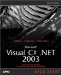Operator Precedence

Say you wanted to add 10 to 15 and multiply the result by 4; you might use this expression:

10 + 15 * 4

And you might expect the result to be 100. Unfortunately, C# will give you a value of 70. What happened ? Instead of adding 10 to 15 and multiplying the result by 4, C# performed the multiplication first, multiplying 15 and 4 and adding the result (60) to 10 to get 70. That's because multiplication has higher precedence than addition, so the multiplication operation in this example was performed first. You can see the C# operator precedence in Table 1.8, from highest to lowest .

Table 1.8. C# Operators Precedence from Highest to Lowest

CATEGORY

OPERATORS

Primary

x.y f(x) a[x] x++ x-- new typeof checked unchecked

Unary

+ - ! ~ ++x --x (T)x

Multiplicative

* / %

+ -

Shift

<< >>

Relational and type testing

< > <= >= is as

Equality

== !=

Logical AND

&

Logical XOR

^

Logical OR

Conditional AND

&&

Conditional OR

Conditional

?:

Assignment

= *= /= %= += -= <<= >>= &= ^= =

You can force C# to ignore the rules of precedence by using parentheses; for example, in the expression (10 + 15) * 4, the addition is performed first, followed by the multiplication, giving you the expected result of 100.

The next step in C# programming is the use of conditional statements , also called branching statements , which let you make decisions and execute code according to the results of those decisions. There are two conditional statements in C#the if statement and the switch statement.Microsoft Visual C#.NET 2003 Kick Start
ISBN: 0672325470
EAN: 2147483647
Year: 2002
Pages: 181

Similar book on Amazon